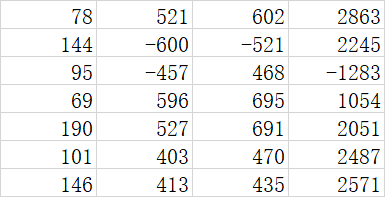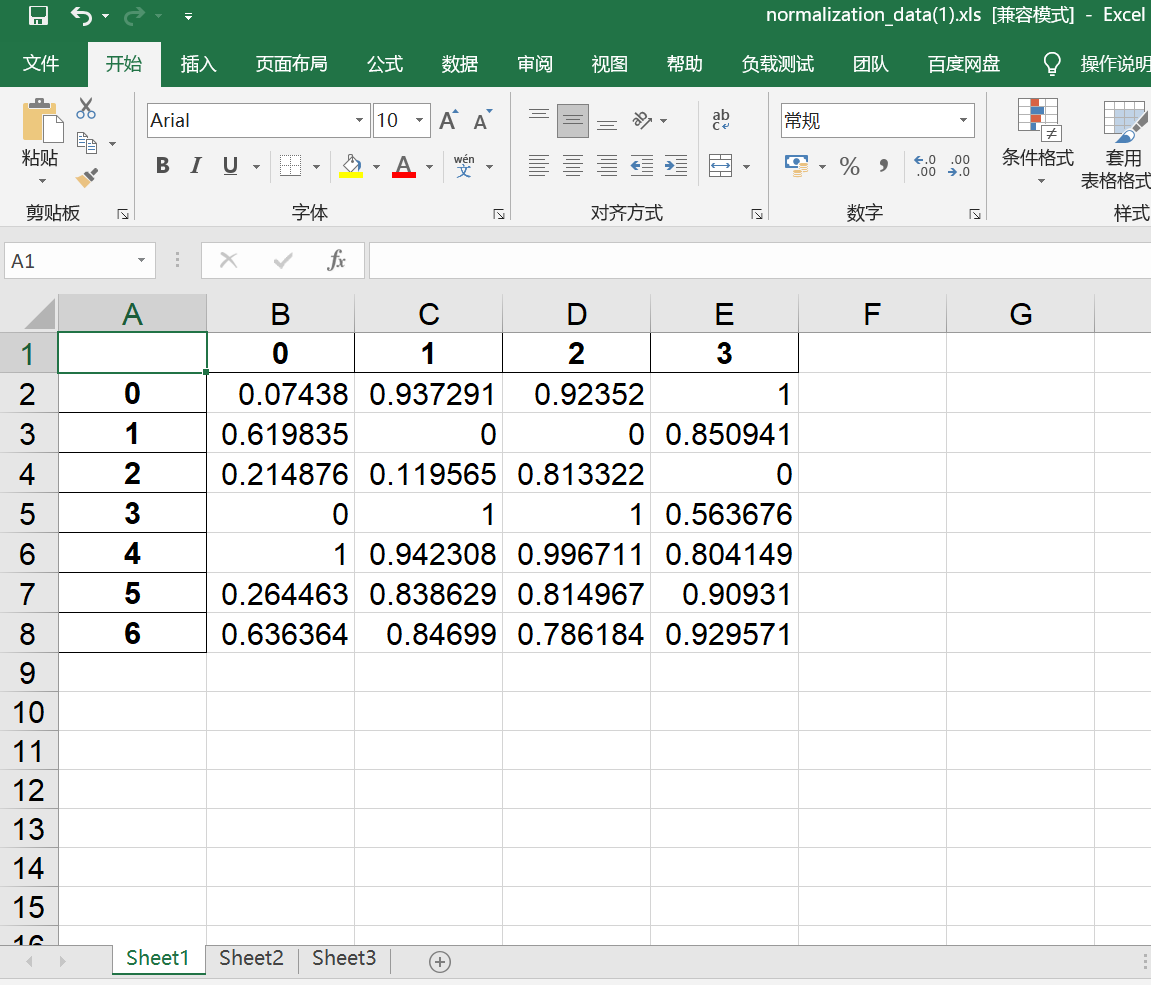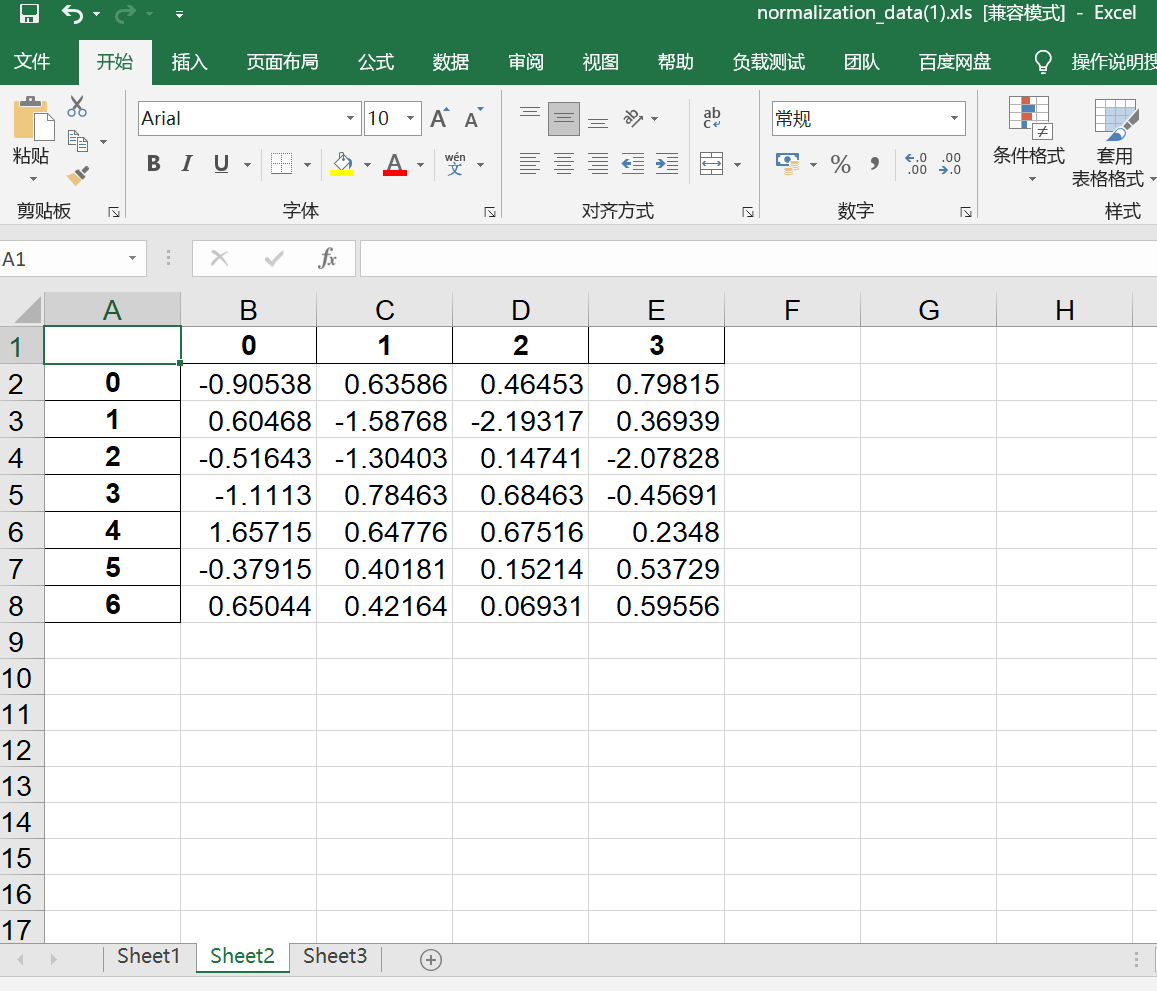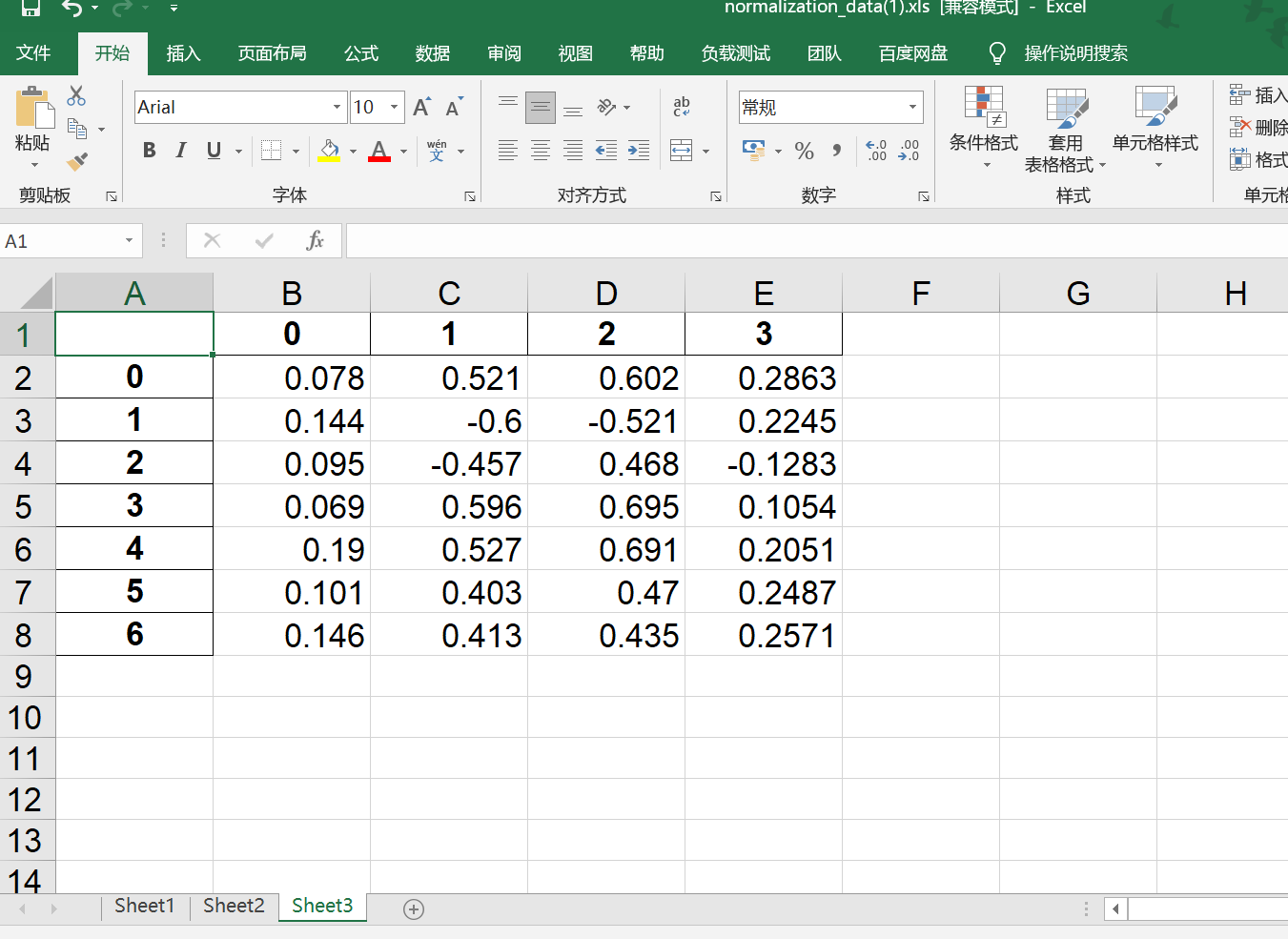• 关于python如何写入excel，网上的资料很多，一般会用xlwt模块，但该模块对于处理xls格式的文件很好，但对于xlsx的文件则会出问题。现在的excel大部分都是xlsx格式，所以我还是推荐大家用xlsxwriter模块。 模块的...
关于python如何写入excel，网上的资料很多，一般会用xlwt模块，但该模块对于处理xls格式的文件很好，但对于xlsx的文件则会出问题。现在的excel大部分都是xlsx格式，所以我还是推荐大家用xlsxwriter模块。
模块的安装很容易，不多讲，下面给出一段示例代码
def data_write(file_path, datas):
f = xlsxwriter.Workbook(file_path)
sheet1 = f.add_worksheet()  # 创建sheet

# 将数据写入第 i 行，第 j 列
i = 0
j=0
while i < len(datas):
sheet1.write(i, j, datas[i])
i+=1
f.close()  #

作为对照，看一段网上找的xlrd模块的处理方法
def data_write(file_path, datas):
f = xlwt.Workbook()
sheet1 = f.add_sheet(u'sheet1',cell_overwrite_ok=True) #创建sheet

#将数据写入第 i 行，第 j 列
i = 0
for data in datas:
for j in range(len(data)):
sheet1.write(i,j,data[j])
i = i + 1

f.save(file_path) #保存文件

可以对比看出两个模块在处理上还是有一些差别，其中file_path是文件路径，datas是一个列表，模块的功能是将列表读入到excel中。


展开全文• 0. 前言Python Data Analysis Library 或 pandas 是基于NumPy 的一种工具，该工具是为了解决数据分析任务而创建...本文介绍如何用pandas读写excel。1. 读取excel读取excel主要通过read_excel函数实现，除了pandas还...
0. 前言Python Data Analysis Library 或 pandas 是基于NumPy 的一种工具，该工具是为了解决数据分析任务而创建的。Pandas 纳入了大量库和一些标准的数据模型，提供了高效地操作大型数据集所需的工具，能使我们快速便捷地处理数据。本文介绍如何用pandas读写excel。1. 读取excel读取excel主要通过read_excel函数实现，除了pandas还需要安装第三方库xlrd。pd.read_excel(io, sheetname=0, header=0, skiprows=None, skip_footer=0, index_col=None, names=None, parse_cols=None, parse_dates=False, date_parser=None, na_values=None, thousands=None, convert_float=True, has_index_names=None, converters=None, dtype=None, true_values=None, false_values=None, engine=None, squeeze=False, **kwds)"""该函数主要的参数为io、sheetname、header、names、encoding。io:excel文件，可以是文件路径、文件网址、file-like对象、xlrd workbook;sheetname:返回指定的sheet，参数可以是字符串（sheet名）、整型（sheet索引）、list（元素为字符串和整型，返回字典{"key":"sheet"}）、none（返回字典，全部sheet）;header:指定数据表的表头，参数可以是int、list of ints，即为索引行数为表头;names:返回指定name的列，参数为array-like对象。encoding:关键字参数，指定以何种编码读取。该函数返回pandas中的DataFrame或dict of DataFrame对象，利用DataFrame的相关操作即可读取相应的数据。"""#代码示例:import pandas as pdexcel_path = "example.xlsx"d = pd.read_excel(excel_path, sheetname=None)print(d["sheet1"].example_column_name)2. 写入excel写入excel主要通过pandas构造DataFrame，调用to_excel方法实现。DataFrame.to_excel(excel_writer, sheet_name="Sheet1", na_rep="", float_format=None, columns=None, header=True, index=True, index_label=None, startrow=0, startcol=0, engine=None, merge_cells=True, encoding=None, inf_rep="inf", verbose=True, freeze_panes=None)"""该函数主要参数为:excel_writer。excel_writer:写入的目标excel文件，可以是文件路径、ExcelWriter对象;sheet_name:被写入的sheet名称，string类型，默认为"sheet1";na_rep:缺失值表示，string类型;header:是否写表头信息，布尔或list of string类型，默认为True;index:是否写行号，布尔类型，默认为True;encoding:指定写入编码，string类型。"""import pandas as pdwriter = pd.ExcelWriter("output.xlsx")df1 = pd.DataFrame(data={"col1":[1,1], "col2":[2,2]})df1.to_excel(writer,"Sheet1")writer.save()
展开全文• 目前用的openpyxl，从数据库获取8W行的数据通过openpyxl写入excel，要花费接近8分钟，这也太慢了，用kettle的插件秒进，python有什么方法能提升速度么，或者openpyxl能批量插入么，按行效率太低了#!/usr/bin/python#...
目前用的openpyxl，从数据库获取8W行的数据通过openpyxl写入excel，要花费接近8分钟，这也太慢了，用kettle的插件秒进，python有什么方法能提升速度么，或者openpyxl能批量插入么，按行效率太低了#!/usr/bin/python# -*- coding: UTF-8 -*-from openpyxl import Workbook as wbookdef xlsx(filename, rows_info, sheet='Result'):if filename and sheet:wb = wbook()_sheet = wb.active_sheet.title = sheetrow = _sheet.max_rowfor line in rows_info:if isinstance(line, str):row_list = [line]elif isinstance(line, dict):row_list = list(line.values())else:try:row_list = list(line)except:row_list = []for col in range(0, len(row_list)):col_info = row_list[col]_sheet.cell(row, col + 1, col_info)row += 1wb.save(filename)else:return '文件和sheet不能为空'
展开全文• 如何python将数据存入同一个excel文件的多张sheet表单 ** 使用to_excel()保存表单时发生覆盖 本想把三个数据表格保存到同一个excel的三个表单，只写了这三行 d1.to_excel(outputfile,sheet_name='Sheet1') d2.to_...
如何用python将数据存入同一个excel文件的多张sheet表单?
使用to_excel()保存表单时发生覆盖:
本想把三个数据表格保存到同一个excel的三个表单，只写了这三行
d1.to_excel(outputfile,sheet_name='Sheet1') d2.to_excel(outputfile,sheet_name='Sheet2') d3.to_excel(outputfile,sheet_name='Sheet3')
写完后打开excel发现只有最后一张表格被保存，前面两张都被覆盖了。
解决方法：

先定义需要存入的excel路径，例如下面的outputfile为excel路径。路径前面加pd.ExcelWriter()括号里面写路径名。
计算你需要的数据，比如我想计算d1,d2,d3，并且最后分别把这三个放入名字为normalization_data.xls的excel中的sheet1,sheet2,sheet33张表单。这里的sheet1,sheet2,sheet3可以是任意的名字，比如也可以叫A,B,C或者其他的。
计算完成后，给每个数据都给使用DataFrame()，例如我写的d1=pd.DataFrame(d1)
给每个数据都使用.to_excel('excel名称'，sheet_name='表单名称')
最后对excel进行保存和关闭outputfile.save()和outputfile.close()

注意事项

写代码时候不一定一次就运行成功，每次运行时要把之前的excel表格关掉再运行，成功后再打开，否则无法写入。

import pandas as pd
import numpy as np

datafile='E:/计算机/数据挖掘/20210421/normalization_data.xls'#参数初始化
outputfile=pd.ExcelWriter('E:/计算机/数据挖掘/20210421/normalization_data(1).xls')#输出地址
data=pd.read_excel(datafile,header=None)#读取数据

#计算
d1=(data-data.min())/(data.max()-data.min())#min-max规范化
d2=(data-data.mean())/data.std()#零-均值规范化
d3=data/10**np.ceil(np.log10(data.abs().max()))#小数定标规范化

#保存
d1=pd.DataFrame(d1)
d2=pd.DataFrame(d2)
d3=pd.DataFrame(d3)
d1.to_excel(outputfile,sheet_name='Sheet1')
d2.to_excel(outputfile,sheet_name='Sheet2')
d3.to_excel(outputfile,sheet_name='Sheet3')
outputfile.save()
outputfile.close()

#成功提示
print("success!")

normalization_data.xls内容如下：保存结果展示：展开全文数据挖掘
• 主要介绍了PYTHON如何读取和写入EXCEL里面的数据,文中通过示例代码介绍的非常详细，对大家的学习或者工作具有一定的参考学习价值,需要的朋友可以参考下
• 最近做了一项工作需要把处理的数据写入Excel表格中进行保存，所以在此就简单介绍使用Python如何把数据保存到excel表格中。数据导入之前需要安装 xlwt依赖包，安装的方法就很简单，直接 pip install xlwt ，如果...
• /usr/bin/python#coding=utf-8#==============================================================================##Filename: demo.py#Description: excel operat#Created: Tue Apr 25 17:10:33 CST 2017#Auth...
• 最近做了一项工作需要把处理的数据写入Excel表格中进行保存，所以在此就简单介绍使用Python如何把数据保存到excel表格中。数据导入之前需要安装 xlwt依赖包，安装的方法就很简单，直接 pip install xlwt ，如果...
• 一、安装xlrd模块到python官网下载二、使用介绍1、导入模块import xlrd2、打开Excel文件读取数据data = xlrd.open_workbook('excelFile.xls')3、使用技巧获取一个工作表table = data.sheets() #通过索引顺序获取...
• python pandas 如何读取Excel数据并重新按照指定格式写入Excel文件呢？ 说一下个人常见的用法心得： 常用且实用的方法： (注意：使用下列方法，记得导入如下库) import pandas as pd import ast 1.读取Excel...
• openpyxl 提供了写入 Excel 的方法，这样我们就可以通过 Python 程序来创建或编辑 Excel 文件啦O(∩_∩)O哈哈~ 1 创建与保存文件 import openpyxl # 创建 wb = openpyxl.Workbook() print('sheetnames = ' + str(wb...
• 需要将字符串写入Excel中，并让该字符串在Excel显示为日期格式。 1、将字符串写入Excel中，格式为“yyyy/m/d” 2、再设置该字符串所在单元格的样式为水平居中、垂直居中 具体用法 import datetime from openpyxl ...
• 我正在脑力激荡的过程中如何最好地解决以下问题.任何输入都非常感谢.Excel工作表列示例：Column A | Column B | Column CApple | Apple |Orange | Orange |Pear | Banana |我希望Excel告诉我A列和B列中的项目是否...
• _, str(t1-t0)) ) return result return function_timer 使用方式的话，就是在要监控的函数定义上面加上 @fn_timer 就行了 以上这篇解决python执行较大excel文件openpyxl慢问题就是小编分享给大家的全部内容了，希望...
• 前言 本文的文字及图片来源于网络,仅供学习、交流使用,不具有任何商业用途,如有问题请及时联系我们以作处理。 PS：如有需要Python学习资料的小伙伴可以加点击下方链接自行获取...又如何使用Python直接往Excel写入
• **任务描述：** 最近在做一个为公文写作提供素材的任务...（此问题转换为：如何将结果存入已存在的excel中？） **xlutils模块** 该模块的功能是作为xlrd和xlwt的桥梁，解决了xlrd中book对象无法编辑的问题。通...json
• UnicodeDecodeError: 'ascii' codec can't decode byte 0xe6 in position 0: ordinal not in range(128)Program ended with exit code: 1当字符串中含有中文的时候，输出到文件则会报错。这时候只需要将带有中文的...
• 最近在做erp项目，其中用到了Excel模版的下载和前后台文件的交互传输 ...python包：xlrd（读取Excel模块），xlwt（写入Excel模块） # coding:utf-8 import xlrd import xlwt """读...
• /usr/bin/env python # -*- coding: utf-8 -*- # import numpy as np import pandas as pd def ColorsSave(colours,name): data = pd.DataFrame(colours) writer = pd.ExcelWriter("F:/find_color/texture ...numpy
• 常用的库是 python-excel 系列： xlrd、xlwt、xlutils、openpyxl xlrd － 读取 Excel 文件 xlwt － 写入 Excel 文件 xlutils － 操作 Excel 文件的实用工具，如复制、分割、筛选等 openpyxl－ ......

# python如何写入excelpython 订阅